# Mean State

Period Mean (original grids) [Watt m-2]
Model Period Mean (intersection) [Watt m-2]
Model Period Mean (complement) [Watt m-2]
Benchmark Period Mean (intersection) [Watt m-2]
Benchmark Period Mean (complement) [Watt m-2]
Bias [Watt m-2]
RMSE [Watt m-2]
Phase Shift [months]
Bias Score 
RMSE Score 
Seasonal Cycle Score 
Spatial Distribution Score 
Interannual Variability Score 
Overall Score 
Benchmark [-] -56.8
CLM5PHSOFF [-] -66.4 -66.5 0.00 -57.0 -52.3 -9.72 16.8 1.70 0.45 0.45 0.75 0.98 0.84 0.65
CLM5PHSON [-] -66.5 -66.5 0.00 -57.0 -52.3 -9.56 16.5 1.67 0.44 0.46 0.76 0.97 0.82 0.65
Period Mean (original grids) [Watt m-2]
Model Period Mean (intersection) [Watt m-2]
Model Period Mean (complement) [Watt m-2]
Benchmark Period Mean (intersection) [Watt m-2]
Benchmark Period Mean (complement) [Watt m-2]
Bias [Watt m-2]
RMSE [Watt m-2]
Phase Shift [months]
Bias Score 
RMSE Score 
Seasonal Cycle Score 
Spatial Distribution Score 
Interannual Variability Score 
Overall Score 
Benchmark [-] -87.0
CLM5PHSOFF [-] -97.6 -98.2 0.00 -87.4 -58.4 -10.6 21.8 0.276 0.67 0.61 0.97 0.74 0.75 0.72
CLM5PHSON [-] -97.3 -98.0 0.00 -87.4 -58.4 -10.2 22.1 0.309 0.68 0.59 0.97 0.81 0.72 0.73
Period Mean (original grids) [Watt m-2]
Model Period Mean (intersection) [Watt m-2]
Model Period Mean (complement) [Watt m-2]
Benchmark Period Mean (intersection) [Watt m-2]
Benchmark Period Mean (complement) [Watt m-2]
Bias [Watt m-2]
RMSE [Watt m-2]
Phase Shift [months]
Bias Score 
RMSE Score 
Seasonal Cycle Score 
Spatial Distribution Score 
Interannual Variability Score 
Overall Score 
Benchmark [-] -81.4
CLM5PHSOFF [-] -92.2 -92.5 0.00 -82.3 -62.6 -10.2 20.4 0.732 0.57 0.53 0.91 0.97 0.73 0.71
CLM5PHSON [-] -91.9 -92.0 0.00 -82.3 -62.6 -7.58 19.7 0.810 0.62 0.51 0.90 0.97 0.71 0.70
Period Mean (original grids) [Watt m-2]
Model Period Mean (intersection) [Watt m-2]
Model Period Mean (complement) [Watt m-2]
Benchmark Period Mean (intersection) [Watt m-2]
Benchmark Period Mean (complement) [Watt m-2]
Bias [Watt m-2]
RMSE [Watt m-2]
Phase Shift [months]
Bias Score 
RMSE Score 
Seasonal Cycle Score 
Spatial Distribution Score 
Interannual Variability Score 
Overall Score 
Benchmark [-] -37.2
CLM5PHSOFF [-] -39.4 -40.3 0.00 -36.9 -41.0 -3.45 16.2 1.16 0.71 0.41 0.85 0.96 0.88 0.71
CLM5PHSON [-] -39.5 -39.6 0.00 -36.9 -41.0 -3.38 16.5 1.11 0.72 0.40 0.86 0.97 0.88 0.70
Period Mean (original grids) [Watt m-2]
Model Period Mean (intersection) [Watt m-2]
Model Period Mean (complement) [Watt m-2]
Benchmark Period Mean (intersection) [Watt m-2]
Benchmark Period Mean (complement) [Watt m-2]
Bias [Watt m-2]
RMSE [Watt m-2]
Phase Shift [months]
Bias Score 
RMSE Score 
Seasonal Cycle Score 
Spatial Distribution Score 
Interannual Variability Score 
Overall Score 
Benchmark [-] -44.6
CLM5PHSOFF [-] -48.1 -48.2 0.00 -43.2 -49.2 -5.03 10.2 1.87 0.36 0.34 0.72 0.97 0.78 0.58
CLM5PHSON [-] -49.7 -49.7 0.00 -43.2 -49.2 -5.81 10.4 1.88 0.35 0.34 0.72 0.98 0.78 0.59
Period Mean (original grids) [Watt m-2]
Model Period Mean (intersection) [Watt m-2]
Model Period Mean (complement) [Watt m-2]
Benchmark Period Mean (intersection) [Watt m-2]
Benchmark Period Mean (complement) [Watt m-2]
Bias [Watt m-2]
RMSE [Watt m-2]
Phase Shift [months]
Bias Score 
RMSE Score 
Seasonal Cycle Score 
Spatial Distribution Score 
Interannual Variability Score 
Overall Score 
Benchmark [-] -42.1
CLM5PHSOFF [-] -53.4 -53.4 0.00 -41.9 -51.7 -11.4 16.2 0.732 0.50 0.51 0.92 1.0 0.89 0.72
CLM5PHSON [-] -52.1 -52.1 0.00 -41.9 -51.7 -10.1 15.7 0.716 0.54 0.49 0.92 0.99 0.89 0.72
Period Mean (original grids) [Watt m-2]
Model Period Mean (intersection) [Watt m-2]
Model Period Mean (complement) [Watt m-2]
Benchmark Period Mean (intersection) [Watt m-2]
Benchmark Period Mean (complement) [Watt m-2]
Bias [Watt m-2]
RMSE [Watt m-2]
Phase Shift [months]
Bias Score 
RMSE Score 
Seasonal Cycle Score 
Spatial Distribution Score 
Interannual Variability Score 
Overall Score 
Benchmark [-] -67.1
CLM5PHSOFF [-] -73.2 -73.0 0.00 -67.3 -59.4 -5.77 16.6 0.982 0.64 0.51 0.86 0.99 0.80 0.72
CLM5PHSON [-] -72.9 -72.6 0.00 -67.3 -59.4 -4.47 16.3 1.02 0.68 0.50 0.85 0.99 0.81 0.72
Period Mean (original grids) [Watt m-2]
Model Period Mean (intersection) [Watt m-2]
Model Period Mean (complement) [Watt m-2]
Benchmark Period Mean (intersection) [Watt m-2]
Benchmark Period Mean (complement) [Watt m-2]
Bias [Watt m-2]
RMSE [Watt m-2]
Phase Shift [months]
Bias Score 
RMSE Score 
Seasonal Cycle Score 
Spatial Distribution Score 
Interannual Variability Score 
Overall Score 
Benchmark [-] -71.2
CLM5PHSOFF [-] -79.3 -79.8 0.00 -72.6 -53.4 -7.30 18.8 0.828 0.64 0.56 0.89 0.96 0.72 0.72
CLM5PHSON [-] -80.3 -80.5 0.00 -72.6 -53.4 -5.12 18.3 0.861 0.68 0.55 0.89 0.95 0.71 0.72
Period Mean (original grids) [Watt m-2]
Model Period Mean (intersection) [Watt m-2]
Model Period Mean (complement) [Watt m-2]
Benchmark Period Mean (intersection) [Watt m-2]
Benchmark Period Mean (complement) [Watt m-2]
Bias [Watt m-2]
RMSE [Watt m-2]
Phase Shift [months]
Bias Score 
RMSE Score 
Seasonal Cycle Score 
Spatial Distribution Score 
Interannual Variability Score 
Overall Score 
Benchmark [-] -31.1
CLM5PHSOFF [-] -39.3 -39.4 0.00 -31.1 -32.1 -8.25 16.1 0.832 0.60 0.44 0.92 0.95 0.90 0.71
CLM5PHSON [-] -38.9 -39.0 0.00 -31.1 -32.1 -7.87 16.1 0.817 0.61 0.43 0.92 0.96 0.90 0.71
Period Mean (original grids) [Watt m-2]
Model Period Mean (intersection) [Watt m-2]
Model Period Mean (complement) [Watt m-2]
Benchmark Period Mean (intersection) [Watt m-2]
Benchmark Period Mean (complement) [Watt m-2]
Bias [Watt m-2]
RMSE [Watt m-2]
Phase Shift [months]
Bias Score 
RMSE Score 
Seasonal Cycle Score 
Spatial Distribution Score 
Interannual Variability Score 
Overall Score 
Benchmark [-] -96.8
CLM5PHSOFF [-] -97.1 -97.3 0.00 -99.4 -63.9 2.03 15.8 0.495 0.67 0.55 0.93 0.95 0.76 0.73
CLM5PHSON [-] -99.6 -99.6 0.00 -99.4 -63.9 3.14 15.8 0.545 0.69 0.54 0.92 0.96 0.74 0.73
Period Mean (original grids) [Watt m-2]
Model Period Mean (intersection) [Watt m-2]
Model Period Mean (complement) [Watt m-2]
Benchmark Period Mean (intersection) [Watt m-2]
Benchmark Period Mean (complement) [Watt m-2]
Bias [Watt m-2]
RMSE [Watt m-2]
Phase Shift [months]
Bias Score 
RMSE Score 
Seasonal Cycle Score 
Spatial Distribution Score 
Interannual Variability Score 
Overall Score 
Benchmark [-] -55.6
CLM5PHSOFF [-] -60.4 -60.3 0.00 -55.1 -60.8 -5.21 11.9 0.722 0.61 0.48 0.91 0.99 0.87 0.72
CLM5PHSON [-] -58.2 -58.2 0.00 -55.1 -60.8 -3.61 11.6 0.745 0.65 0.47 0.91 0.97 0.87 0.72
Period Mean (original grids) [Watt m-2]
Model Period Mean (intersection) [Watt m-2]
Model Period Mean (complement) [Watt m-2]
Benchmark Period Mean (intersection) [Watt m-2]
Benchmark Period Mean (complement) [Watt m-2]
Bias [Watt m-2]
RMSE [Watt m-2]
Phase Shift [months]
Bias Score 
RMSE Score 
Seasonal Cycle Score 
Spatial Distribution Score 
Interannual Variability Score 
Overall Score 
Benchmark [-] -52.6
CLM5PHSOFF [-] -74.4 -70.9 0.00 -65.2 -46.5 -5.75 17.6 0.968 0.55 0.50 0.87 0.95 0.78 0.69
CLM5PHSON [-] -71.2 -71.2 0.00 -65.2 -46.5 -4.70 17.3 0.974 0.58 0.49 0.87 0.94 0.77 0.69
Period Mean (original grids) [Watt m-2]
Model Period Mean (intersection) [Watt m-2]
Model Period Mean (complement) [Watt m-2]
Benchmark Period Mean (intersection) [Watt m-2]
Benchmark Period Mean (complement) [Watt m-2]
Bias [Watt m-2]
RMSE [Watt m-2]
Phase Shift [months]
Bias Score 
RMSE Score 
Seasonal Cycle Score 
Spatial Distribution Score 
Interannual Variability Score 
Overall Score 
Benchmark [-] -121.
CLM5PHSOFF [-] -107. -107. 0.00 -122. -81.3 14.8 25.7 0.671 0.56 0.53 0.91 0.78 0.63 0.66
CLM5PHSON [-] -108. -108. 0.00 -122. -81.3 15.4 25.9 0.675 0.56 0.53 0.91 0.82 0.64 0.66
Period Mean (original grids) [Watt m-2]
Model Period Mean (intersection) [Watt m-2]
Model Period Mean (complement) [Watt m-2]
Benchmark Period Mean (intersection) [Watt m-2]
Benchmark Period Mean (complement) [Watt m-2]
Bias [Watt m-2]
RMSE [Watt m-2]
Phase Shift [months]
Bias Score 
RMSE Score 
Seasonal Cycle Score 
Spatial Distribution Score 
Interannual Variability Score 
Overall Score 
Benchmark [-] -34.3
CLM5PHSOFF [-] -42.9 -42.9 0.00 -34.2 -36.5 -8.74 15.3 0.805 0.57 0.46 0.90 0.83 0.88 0.68
CLM5PHSON [-] -42.1 -42.1 0.00 -34.2 -36.5 -8.40 15.3 0.806 0.58 0.45 0.89 0.84 0.88 0.68
Period Mean (original grids) [Watt m-2]
Model Period Mean (intersection) [Watt m-2]
Model Period Mean (complement) [Watt m-2]
Benchmark Period Mean (intersection) [Watt m-2]
Benchmark Period Mean (complement) [Watt m-2]
Bias [Watt m-2]
RMSE [Watt m-2]
Phase Shift [months]
Bias Score 
RMSE Score 
Seasonal Cycle Score 
Spatial Distribution Score 
Interannual Variability Score 
Overall Score 
Benchmark [-] -70.7
CLM5PHSOFF [-] -82.6 -82.8 0.00 -73.4 -57.5 -9.52 16.8 1.09 0.50 0.48 0.83 0.99 0.81 0.68
CLM5PHSON [-] -84.6 -84.6 0.00 -73.4 -57.5 -7.82 16.0 1.09 0.53 0.48 0.83 1.0 0.80 0.69
Period Mean (original grids) [Watt m-2]
Model Period Mean (intersection) [Watt m-2]
Model Period Mean (complement) [Watt m-2]
Benchmark Period Mean (intersection) [Watt m-2]
Benchmark Period Mean (complement) [Watt m-2]
Bias [Watt m-2]
RMSE [Watt m-2]
Phase Shift [months]
Bias Score 
RMSE Score 
Seasonal Cycle Score 
Spatial Distribution Score 
Interannual Variability Score 
Overall Score 
Benchmark [-] -53.2
CLM5PHSOFF [-] -64.2 -64.1 0.00 -52.9 -58.5 -11.2 14.7 0.750 0.41 0.49 0.88 0.99 0.89 0.69
CLM5PHSON [-] -61.8 -61.7 0.00 -52.9 -58.5 -8.67 13.0 0.738 0.50 0.47 0.88 0.99 0.89 0.70
Period Mean (original grids) [Watt m-2]
Model Period Mean (intersection) [Watt m-2]
Model Period Mean (complement) [Watt m-2]
Benchmark Period Mean (intersection) [Watt m-2]
Benchmark Period Mean (complement) [Watt m-2]
Bias [Watt m-2]
RMSE [Watt m-2]
Phase Shift [months]
Bias Score 
RMSE Score 
Seasonal Cycle Score 
Spatial Distribution Score 
Interannual Variability Score 
Overall Score 
Benchmark [-] -42.5
CLM5PHSOFF [-] -54.2 -54.2 0.00 -42.4 -45.4 -11.8 16.2 0.542 0.50 0.53 0.94 0.99 0.87 0.73
CLM5PHSON [-] -52.8 -52.8 0.00 -42.4 -45.4 -10.2 15.3 0.536 0.55 0.51 0.94 0.98 0.86 0.73
Period Mean (original grids) [Watt m-2]
Model Period Mean (intersection) [Watt m-2]
Model Period Mean (complement) [Watt m-2]
Benchmark Period Mean (intersection) [Watt m-2]
Benchmark Period Mean (complement) [Watt m-2]
Bias [Watt m-2]
RMSE [Watt m-2]
Phase Shift [months]
Bias Score 
RMSE Score 
Seasonal Cycle Score 
Spatial Distribution Score 
Interannual Variability Score 
Overall Score 
Benchmark [-] -80.3
CLM5PHSOFF [-] -88.1 -87.9 0.00 -80.8 -54.9 -7.23 18.1 0.220 0.70 0.58 0.98 0.93 0.77 0.75
CLM5PHSON [-] -86.8 -86.6 0.00 -80.8 -54.9 -5.44 18.1 0.247 0.72 0.57 0.97 0.94 0.77 0.76
Period Mean (original grids) [Watt m-2]
Model Period Mean (intersection) [Watt m-2]
Model Period Mean (complement) [Watt m-2]
Benchmark Period Mean (intersection) [Watt m-2]
Benchmark Period Mean (complement) [Watt m-2]
Bias [Watt m-2]
RMSE [Watt m-2]
Phase Shift [months]
Bias Score 
RMSE Score 
Seasonal Cycle Score 
Spatial Distribution Score 
Interannual Variability Score 
Overall Score 
Benchmark [-] -43.7
CLM5PHSOFF [-] -52.5 -52.4 0.00 -43.6 -47.7 -8.76 13.9 1.67 0.47 0.45 0.77 0.77 0.77 0.61
CLM5PHSON [-] -51.8 -51.7 0.00 -43.6 -47.7 -8.13 13.1 1.56 0.46 0.46 0.79 0.87 0.75 0.63
Period Mean (original grids) [Watt m-2]
Model Period Mean (intersection) [Watt m-2]
Model Period Mean (complement) [Watt m-2]
Benchmark Period Mean (intersection) [Watt m-2]
Benchmark Period Mean (complement) [Watt m-2]
Bias [Watt m-2]
RMSE [Watt m-2]
Phase Shift [months]
Bias Score 
RMSE Score 
Seasonal Cycle Score 
Spatial Distribution Score 
Interannual Variability Score 
Overall Score 
Benchmark [-] -64.5
CLM5PHSOFF [-] -73.7 -74.1 0.00 -64.8 -56.3 -9.33 15.8 0.826 0.58 0.48 0.89 0.90 0.84 0.69
CLM5PHSON [-] -72.2 -72.6 0.00 -64.8 -56.3 -7.17 14.8 0.801 0.65 0.48 0.90 0.90 0.81 0.70
Period Mean (original grids) [Watt m-2]
Model Period Mean (intersection) [Watt m-2]
Model Period Mean (complement) [Watt m-2]
Benchmark Period Mean (intersection) [Watt m-2]
Benchmark Period Mean (complement) [Watt m-2]
Bias [Watt m-2]
RMSE [Watt m-2]
Phase Shift [months]
Bias Score 
RMSE Score 
Seasonal Cycle Score 
Spatial Distribution Score 
Interannual Variability Score 
Overall Score 
Benchmark [-] -80.0
CLM5PHSOFF [-] -85.0 -84.6 0.00 -83.0 -54.0 -1.47 20.5 0.775 0.50 0.44 0.89 0.90 0.77 0.66
CLM5PHSON [-] -85.7 -85.3 0.00 -83.0 -54.0 0.359 19.8 0.785 0.54 0.44 0.89 0.89 0.77 0.66

# Temporally integrated period mean

BENCHMARK MEAN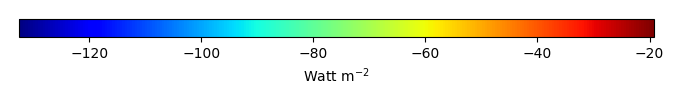MODEL MEANBIAS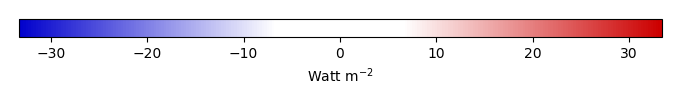BIAS SCORERMSE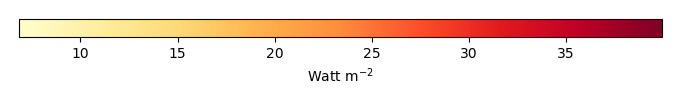RMSE SCOREBENCHMARK INTERANNUAL VARIABILITY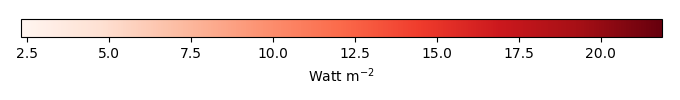MODEL INTERANNUAL VARIABILITYINTERANNUAL VARIABILITY SCOREBENCHMARK MAX MONTHMODEL MAX MONTHDIFFERENCE IN MAX MONTH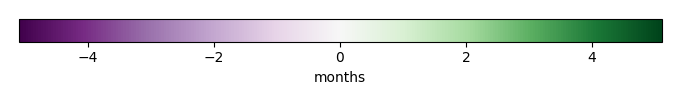SEASONAL CYCLE SCORESPATIAL TAYLOR DIAGRAMMODEL COLORS# Spatially integrated regional mean

MODEL COLORSREGIONAL MEANANNUAL CYCLEMONTHLY ANOMALYANNUAL CYCLE# All Models

BenchmarkCLM5PHSOFFCLM5PHSON# Data Information

creation_date: Tue Jul 1 08:25:00 PDT 2014

source_file: This product is generated from monthly 1 degree GEWEX SRB Radiation observations